﻿ Advanced DNA Mapping Schemes for Exon Prediction Using Digital FiltersAmerican Journal of Biomedical Engineering

p-ISSN: 2163-1050    e-ISSN: 2163-1077

2016;  6(1): 25-31

doi:10.5923/j.ajbe.20160601.04Advanced DNA Mapping Schemes for Exon Prediction Using Digital Filters

Heba Mohamed. Wassfy1, 2, Mustafa M. Abd Elnaby1, Mohamed Labib Salem2, 3, Mai S. Mabrouk4, Abdel-Aziz Awad Zidan2, 3

1Electronics & Electrical Communication Department, Faculty of Engineering, Tanta University, Egypt

2Center of Excellence in Cancer Research (CECR), Tanta University Teaching Hospital, Egypt

3Immunology & Biotechnology Division, Zoology Departments, Faculty of Science, Tanta University, Egypt

4Biomedical Engineering Department, Misr University for Science & Technology, Egypt

Correspondence to: Heba Mohamed. Wassfy, Electronics & Electrical Communication Department, Faculty of Engineering, Tanta University, Egypt.
 Email: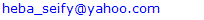Abstract

Genomic signal processing (GSP) is the engineering area concerned with genomic data analysis using digital signal processing techniques by conversion of the genomic sequence into numerical one as a first step. One of the central issues in GSP is maximizing the accuracy of protein coding region prediction in a given DNA sequence. In this study advanced DNA numerical representations (genetic code context, 2-bit binary and EIIP) were compared in terms of their sensitivity, specificity and correlation coefficient for maximizing the accuracy of the prediction of protein coding region. Digital filters based technique has been applied to extract the period 3 components and removing the undesired noise from the DNA sequence. Results from implementation of the technique on 8 human genes showed that the 2-bit binary representation scheme associated with the used filtering technique has the maximum accuracy compared to the other tested schemes. These findings suggests that the 2-bit binary representation scheme greatly enhances the prediction accuracy of the protein coding region using digital filters opening a new avenue to use this scheme in different applications.

Keywords: Digital filters, DNA, bioinformatics, Genomic signal processing

Cite this paper: Heba Mohamed. Wassfy, Mustafa M. Abd Elnaby, Mohamed Labib Salem, Mai S. Mabrouk, Abdel-Aziz Awad Zidan, Advanced DNA Mapping Schemes for Exon Prediction Using Digital Filters, American Journal of Biomedical Engineering, Vol. 6 No. 1, 2016, pp. 25-31. doi: 10.5923/j.ajbe.20160601.04.

1. Introduction

Genomic sequence analysis using digital signal processing (DSP) techniques such as filtering, transformation and data compression has been attracted the attention of researchers in recent years . Digital signal processing is an important area of engineering which comprehends the manipulation of numerically represented signal to produce a higher quality signal than the original one . The impact of DSP tools on genomic sequences is the new field of genomic signal processing (GSP) which can be defined as the spectral analysis of genomic signals by DSP algorithms and techniques to achieve a variety of goals such as gene prediction, identification of hotspot locations in proteins and motif prediction . This leads to deep understanding of the living system for development of new therapeutic and diagnostic tools . Genomic data such as deoxyribonucleic acid DNA is discrete in nature and can be mathematically represented by a permutation of four characters A (Adenine), T (Thymine), C (cytosine), G (guanine) of different lengths . The eukaryotic DNA is divided into genes and intergenic spaces. A gene is divided into two sub-regions called exons and introns as shown in Fig.1.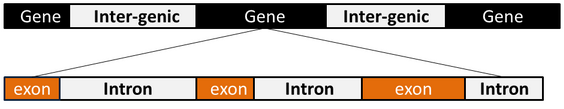Figure 1. DNA structure of eukaryotes
After sequencing of a new organism, the accurate identification of exons and introns boundaries is an essential and critical step to recognize the hidden features and information about its genome  .
In order to apply digital signal processing techniques for prediction of protein coding region (exons) for a given DNA sequence, the character genomic sequence must be converted into numerical sequence .
The existing numerical representation schemes can be classified into three major groups:(i) Fixed mapping methods (FM) in which the DNA nucleotides are transformed into a series of arbitrarily numerical sequences , (ii) Physico chemical property based mapping methods (PCPBM) in which biophysical and biochemical properties of DNA nucleotides are used for DNA mapping  and (iii) Statistical property based mapping methods (SPBM) in which the DNA sequences are mapped in terms of some statistical properties . Previous studies show that Electron Ion Interaction Potential (EIIP)  which is PCPBM based representation scheme is the most accurate mapping method for spectral analysis for DNA sequences as it has the ability to recognize protein coding region in some genomes where the other representations fails to recognize them, Also EIIP reduces the computational efficiency by 75% [9, 10], . Therefore, EIIP is the most widely used representation scheme for prediction of protein coding regions of a given DNA sequence  [4, 13].
There are other advanced DNA representation schemes have been used such as: Genetic code context (GCC) , 2-bit binary . In genetic code context (GCC) representation scheme the DNA sequence is mapped into one dimension indicator sequence according to the composition and distribution of the amino acids in three coding frames . The single strand DNA sequence is translated into the triple codons from three reading frames, then each amino acid is represented by a unique complex number based on the hydrophobicity properties and residue volumes of the amino acids , as these two properties are the main driving forces of amino acids in protein folding. In 2-bit binary representations scheme the DNA sequence represented by 1-dimensional indicator sequence by mapping the nucleotides A, C, G, T into two binary namely, 00, 11, 10, and 01 respectively.
The above illustrated representation schemes are mainly used for prediction of protein coding region using discrete Fourier transform DFT spectral analysis. On the other hand, digital filters have been used for the prediction of protein coding region for EIIP coded sequences of several genes   and the results showed that using of digital filters yields to better prediction accuracy than DFT based approach.
Among various types of DNA numerical representations have been used, it is difficult to choose the appropriate representation scheme as it greatly affects the accuracy, sensitivity and computational efficiency of the technique used.
Our study aims to explore the effect of the other advanced numerical representation schemes (GCC) and the 2-bit binary on the efficiency of the protein coding region prediction using digital filters by measuring the sensitivity, specificity and correlation coefficient of each scheme compared to EIIP representation scheme.

2. Methodology

2.1. DNA Sequence Database

The DNA sequences of several eukaryotic genes were downloaded from HMR195 dataset prepared by Sanga Rogic . As a model genes, eight human genes with various exon numbers were used for measuring the performance of the tested numerical representation schemes. These genes were chosen to satisfy two conditions; First, the sequence length not to exceed 10,000 base pairs and, second, the number of exons should be less than 4 exons to assert accuracy.

2.2. DNA Numerical Representation

The DNA sequences of the selected genes were mapped into the three selected representation schemes as follows:
2.2.1. Genetic Code Context (GCC)
For a given DNA sequence X = ACGATTCAGGT the triple codons for the three reading frames are, ACG CGA GAT ATT TTC TCA CAG AGG GGT. The corresponding encoded amino acids are [T, R, D, I, F, S, Q, R, G]. Then each amino acid is represented by a unique complex number. The real part represents the hydrophobicity index  while the complex part represents the residue volume of each amino acid  as shown in Table (1). Thus the DNA numerical vector is [0.05 + 118.2i, 0.60 + 181.2i, 0.46 + 110.8i, 2.22 + 168.5i, 2.02 + 189.0i, 0.05 + 88.7i, 148.7i, 0.60 + 181.2i, 0.07 + 60.0i].
 Table 1. GCC based numerical representation of the 20 amino acid based on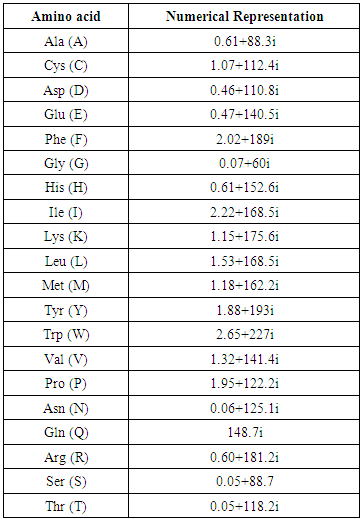2.2.2. (2-bit) Binary Representation
For position i in the DNA sequence X[i] = ACGATTCAGGT, the 2-bit indicator sequence values are defined as, A=00, G=10, T=01, C=11. Thus the corresponding DNA 2-bit numerical sequence is [00, 11, 10, 00, 01, 01, 11, 00, 10, 10, 01].
2.2.3. Electron Ion Interaction Potential (EIIP)
For position i in the DNA sequence X[i] = ACGATTCAGGT, the EIIP indicator sequence values are defined as, A = 0.1260, G = 0.0806, C = 0.1340, T = 0.1335.
Thus the corresponding EIIP numerical sequence is, [0.1260, 0.1340, 0.0806, 0.1260, 0.1335, 0.1335, 0.1340, 0.1260, 0.0806, 0.0806, 0.1335].

2.3. Prediction of Protein Coding Region Using Digital Filter

Infinite impulse response (IIR) digital filter with inverse Chebyshev approximation has been chosen due to its high selectivity which can be achieved with a low order transfer function. Moreover, inverse chebyshev filter doesn't exhibit a ripple in its passband amplitude response  which is highly needed for the prediction of protein coding region application. Zero phase filtering is used to eliminate the IIR filter delay .
Figure (2) describes the steps involved in the realization of the technique using MATLAB, in which the DNA numerical sequence has filtered through narrowband bandpass filter designed to extract the period 3 component, the noise has removed using low pass filter in order to measure the evaluation parameters for each numerical representation scheme.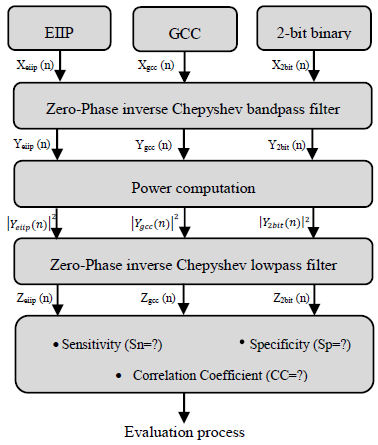Figure 2. Overall scheme of the proposed system
2.3.1. Zero-Phase Bandpass Filtering
The numerical DNA sequence was filtered using Inverse Chepyshev bandpass filter with the following specifications: Filter order N=3, the lower & upper passband edge frequencies [0.663, 0.669], the lower & upper stopband edge frequencies [0.66, 0.672], the maximum passband attenuation=1dB, the minimum stopband attenuation=30dB.
2.3.2. Power Computation
The power of the output filtered was calculated by squaring the signal magnitude.
2.3.3. Zero-Phase Lowpass Filtering
The squared signal was filtered to eliminate the background noise using Inverse Chepyshev lowpass filter with the following specifications: Filter order N=16, Passband edge frequency=0.5, Stopband edge frequency=0.6, the maximum passband attenuation=1dB, the minimum stopband attenuation=80dB.

2.4. Calculation of Evaluation Parameters

The sensitivity, specificity and correlation coefficient were calculated as evaluation parameters to measure the effect of different DNA representation schemes on the overall efficiency of the prediction of protein coding region using digital filter as follows:
Sensitivity (Sn) is the capability of the representation scheme to predict the true exons. In contrast, specificity  is the capability of the representation scheme to exclude the false exons. The DNA representation scheme is considered accurate only if both sensitivity and specificity are high. Correlation coefficient (CC) is the measure of accuracy and ranges from -1 to 1 . Sn, Sp, and CC can be described by the following equations: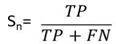(1)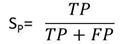(2)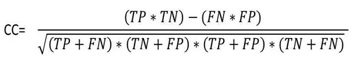(3)
Where:
TP: True Positive, TN: True Negative, FN: False Negative, FP: False Positive, Figure (3) shows the definition of these measures, we have calculated these measures so that, If the peak of an intron region greater than half of the minimum exon peak it will considered as false positive (false exon) otherwise it will considered as true negative.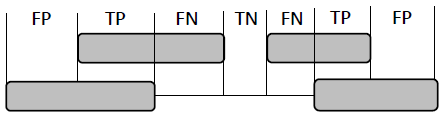Figure 3. Definition of four basic measures of exon prediction accuracy at the nucleotide level

3. Results and Discussion

3.1. Demographic of the Dataset Used

In order to achieve our aim we have applied the prediction technique using IIR inverse Chepyshev digital filter on 8 human testing genes of single and multiple exons downloaded from HMR195 dataset. The accession numbers, sequence length and true exon locations of the genes are shown in Table 2.
 Table 2. Demographic of the dataset used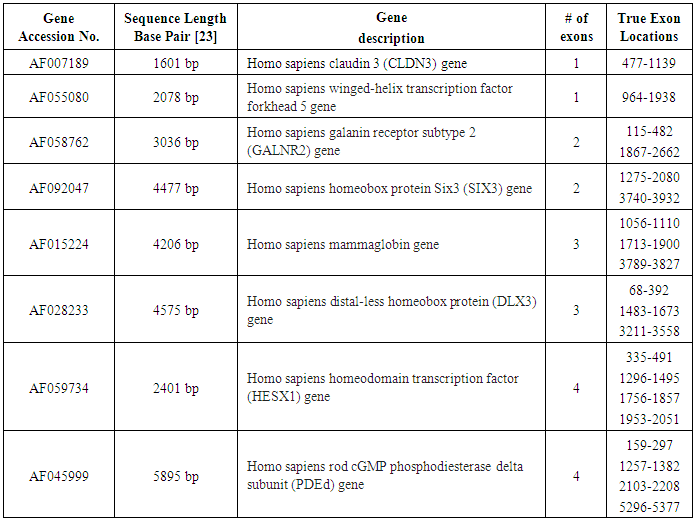Results of two sample genes with various exon numbers are shown in figs 4 and 5. The true exon locations represented by the red dashed box, and the false exons represented by the black bars. When investigating the prediction performance of the different representation schemes on different genes with two exonic regions, we found that, the 2-bit binary representation scheme showed precise results at the level of nucleotide position identification as shown in fig 4 where the 2-bit binary scheme efficiently detect the two true exons of (GALNR2) gene at their right positions (115-482, 1867-2662) without exhibition of false exons. Moreover it showed highest sensitivity, specificity and correlation coefficient values (100, 89.2, .91) respectively compared to other schemes as shown in table 3.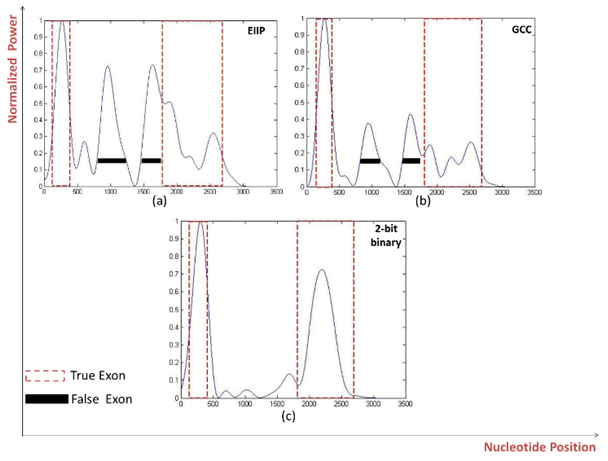Figure 4. Power spectrum of Homo sapiens galanin receptor subtype 2 GALNR2 (AF058762) using a) EIIP b) GCC c) 2-bit binary mapping schemes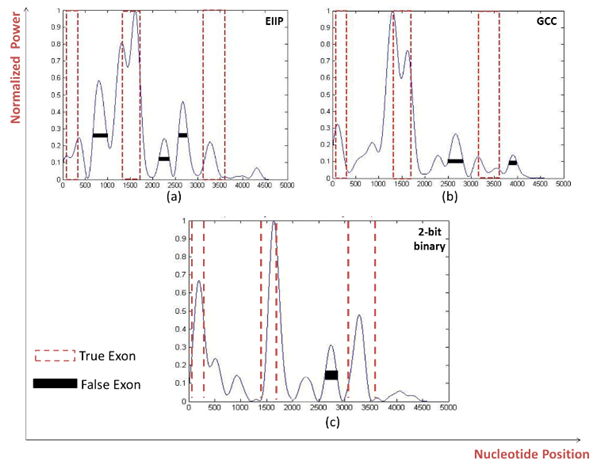Figure 5. Power spectrum of Homo sapiens distal-less homobox protein DLX3 gene (AF028233) using a) EIIP b) GCC c) 2-bit binary mapping schemes
 Table 3. Evaluation parameters for the tested genes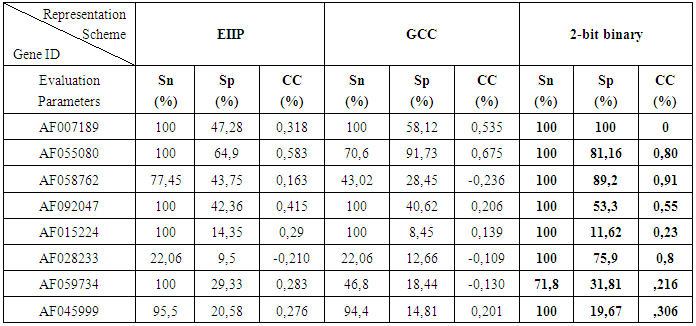When the prediction technique using the three different representation schemes has been applied on different genes of three exonic regions, the results showed that the 2-bit binary representation scheme clearly enhanced the prediction accuracy compared to all other representation scheme as shown in fig 5 where the distal-less homobox protein DLX3 gene (AF028233) is analyzed, the true nucleotide positions were accurately detected by using the 2-bit binary representation scheme with minimum number of false exons which consistent with the highest levels of sensitivity, specificity and correlation coefficient achieved by this scheme (100%, 75,9%, 0.8) respectively as shown in table 3.

4. Conclusions

To explore the effect of using different DNA numerical representation schemes on the accuracy improvement of the prediction of protein coding regions in a given eukaryotic DNA sequence, Digital filter based prediction technique has been applied on 8 human genes used as benchmark dataset after numerically converting them into three different numerical representation schemes. The results showed that the 2-bit binary representation scheme greatly enhance the prediction accuracy at the level of true nucleotide position identification associated with high levels of sensitivity, specificity and correlation coefficient compared with EIIP and GCC representation schemes. These findings suggest that the 2-bit binary is an effective representation scheme for prediction of protein coding regions of unknown target sequences using digital filters opening a new avenue to use this scheme in different applications.

ACKNOWLEDGMENTS

This work has been supported by a grant (ID# 5245) funded from the Science and Technology Development Fund (STDF), Ministry of Scientific Research, Egypt to Mohamed L. Salem, the Principal investigator of this project.

References

  Akhtar, M., J. Epps, and E. Ambikairajah, Signal processing in sequence analysis: advances in eukaryotic gene prediction. Selected Topics in Signal Processing, IEEE Journal of, 2008. 2(3): p. 310-321.  Andreas, A., Digital signal processing: Signals, systems, and filters. 2006, McGraw-Hill, New York.  Anastassiou, D., Genomic signal processing. Signal Processing Magazine, IEEE, 2001. 18(4): p. 8-20.  Inbamalar, T. and R. Sivakumar, Study of DNA sequence analysis using DSP Techniques‖. Journal of Automation and Control Engineering Vol, 2013. 1(4).  Alberts, B., et al., Essential cell biology. 2013: Garland Science.  Mabrouk, M., S.,“A Study of the Potential of EIIP Mapping Method in Exon Prediction Using the Frequency Domain Techniques,”. American Journal of Biomedical Engineering, 2012. 2(2): p. 17-22.  bai Arniker, S. and H.K. Kwan. Advanced numerical representation of DNA sequences. in International Conference on Bioscience, Biochemistry and Bioinformatics IPCBEE. 2012.  Cosic, I., Macromolecular bioactivity: is it resonant interaction between macromolecules?-theory and applications. Biomedical Engineering, IEEE Transactions on, 1994. 41(12): p. 1101-1114.  Lazović, J., Selection of amino acid parameters for Fourier transform-based analysis of proteins. Computer applications in the biosciences: CABIOS, 1996. 12(6): p. 553-562.  Ramachandran, P. and A. Antoniou, Identification of hot-spot locations in proteins using digital filters. Selected Topics in Signal Processing, IEEE Journal of, 2008. 2(3): p. 378-389.  Abo-Zahhad, M., S.M. Ahmed, and S.A. Abd-Elrahman, Integrated Model of DNA Sequence Numerical Representation and Artificial Neural Network for Human Donor and Acceptor Sites Prediction. International Journal of Information Technology and Computer Science (IJITCS), 2014. 6(8): p. 51.  Ramachandran, P., W.-S. Lu, and A. Antoniou. Location of exons in DNA sequences using digital filters. in Circuits and Systems, 2009. ISCAS 2009. IEEE International Symposium on. 2009. IEEE.  Saberkari, H., et al. Prediction of protein coding regions in DNA sequences using signal processing methods. in Industrial Electronics and Applications (ISIEA), 2012 IEEE Symposium on. 2012. IEEE.  Kwan, H.K. and S.B. Arniker. Numerical representation of DNA sequences. in Electro/Information Technology, 2009. eit'09. IEEE International Conference on. 2009. IEEE.  Ranawana, R. and V. Palade, A neural network based multi-classifier system for gene identification in DNA sequences. Neural Computing & Applications, 2005. 14(2): p. 122-131.  Yin, C. and S.S.-T. Yau. Numerical representation of DNA sequences based on genetic code context and its applications in periodicity analysis of genomes. in Computational Intelligence in Bioinformatics and Computational Biology, 2008. CIBCB'08. IEEE Symposium on. 2008. IEEE.  Goldsack, D. and R. Chalifoux, Contribution of the free energy of mixing of hydrophobic side chains to the stability of the tertiary structure of proteins. Journal of theoretical biology, 1973. 39(3): p. 645-651.  Vaidyanathan, P. and B.-J. Yoon. Digital filters for gene prediction applications. in Signals, Systems and Computers, 2002. Conference Record of the Thirty-Sixth Asilomar Conference on. 2002. IEEE.  Rogic, S., A.K. Mackworth, and F.B. Ouellette, Evaluation of gene-finding programs on mammalian sequences. Genome research, 2001. 11(5): p. 817-832.  Argos, P., J. Rao, and P.A. Hargrave, Structural prediction of membrane-bound proteins. Eur. J. Biochem, 1982. 128(2-3): p. 565-575.  Chakravarthy, N., et al., Autoregressive modeling and feature analysis of DNA sequences. EURASIP Journal on Applied Signal Processing, 2004. 2004: p. 13-28.  Burset, M. and R. Guigo, Evaluation of gene structure prediction programs. Genomics, 1996. 34(3): p. 353-367.  Press, W., et al., Statistical description of data. Numerical Recipes in C: The Art of Scientific Computing, 1992: p. 636.